Courses

# RD Sharma Solutions Ex-2.2, (Part - 2), Exponents Of Real Numbers, Class 9, Maths Class 9 Notes | EduRev

## Class 9 : RD Sharma Solutions Ex-2.2, (Part - 2), Exponents Of Real Numbers, Class 9, Maths Class 9 Notes | EduRev

The document RD Sharma Solutions Ex-2.2, (Part - 2), Exponents Of Real Numbers, Class 9, Maths Class 9 Notes | EduRev is a part of the Class 9 Course RD Sharma Solutions for Class 9 Mathematics.
All you need of Class 9 at this link: Class 9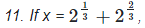show that x3 − 6x = 6

x− 6x = 6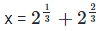Putting cube on both the sides, we get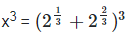As we know, (a+b)3 = a3 + b3 + 3ab(a+b)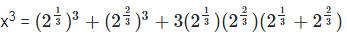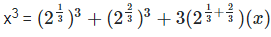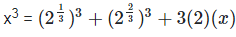x3 = 6 + 6x

x3 – 6x = 6

Hence proved

12. Determine (8x)x, if 9x+2 = 240 + 9x.

9x+2 = 240 + 9x

9x .92 = 240 + 9x

Let 9x be y

81y = 240 + y

81y – y = 240

80y = 240

y = 3

Since, y = 3

Then,

9x = 3

32x = 3

Therefore, x = 1/2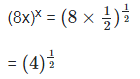= 2

Therefore (8x)x = 2

13. If 3x+1 = 9x-2, find the value of 21+x

3x+1 = 9x-2

3x+1 = 32x-4

x + 1 = 2x – 4

x = 5

Therefore the value of 21+x = 21+5 = 26 = 64

14. If 34x = (81)-1 and (10)1/y = 0.0001, find the value of 2-x+4y.

34x = (81)-1 and (10)1/y = 0.0001

34x = (3)-4

x = -1

And (10)1/y = 0.0001

(10)1/y = (10)−4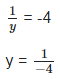To find the value of 2-x+4y, we need to substitute the value of x and y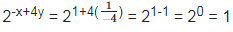15. If 53x = 125 and 10y = 0.001. Find x and y.

53x = 125 and 10y = 0.001

53x = 53

x = 1

Now,

10y = 0.001

10y = 10-3

y = -3

Therefore, the value of x = 1 and the value of y = -3

16. Solve the following equations

(i) 3x+1 = 27×34

3x+1 = 33×34

3x+1 = 33+4

x + 1 = 3 + 4          [By equating exponents]

x + 1 = 7

x = 7 − 1

x = 6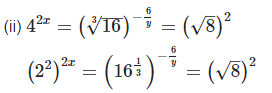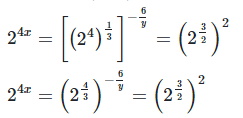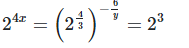24x = 23

4x = 3  (By equating exponents)

x = 3/4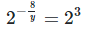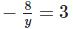(By equating exponents)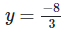(iii). 3x−1×52y−3 = 225

3x−1×52y−3 = 32×52

x−1 = 2   [By equating exponents]

x = 3

3x−1×52y−3 = 32×52

2y−3 = 2   [By equating exponents]

2y = 5

y = 5/2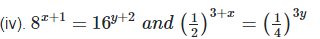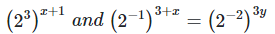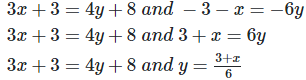3x+3 = 4y+8  —eq1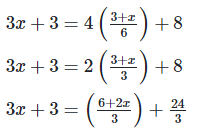3(3x+3) = 6+2x+24

9x+9 = 30+2x

7x = 21

x = 21/7

x = 3

Putting value of x in eq2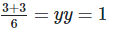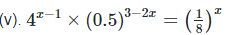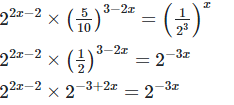2x−2−3+2x = −3x  [By equating exponents]

4x+3x = 5

7x = 5

x = 5/7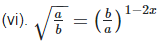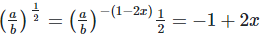[By equating exponents]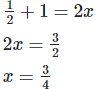17. If a and b are distinct positive primes such that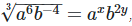find x and y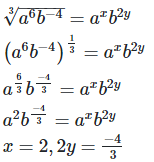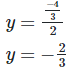18.If a and b are different positive primes such that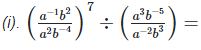axby find x and y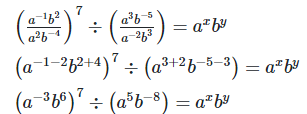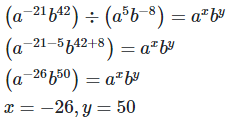(ii) (a+b)−1(a−1+b−1) = axby,find x and y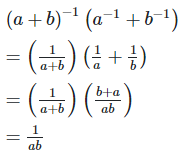=(ab)−1 = a−1b−1

By equating exponents

x = −1,y = −1

Therefore x+y+2 = −1−1+2 = 0

19. If 2x×3y×5z = 2160 , find x,y and z. Hence compute the value of 3x×2−y×5−z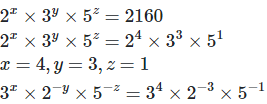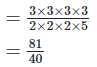20. If 1176 = 2a×3b×7c, find the values of a,b and c. Hence compute the value of 2a×3b×7−c as a fraction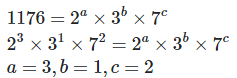We have to find the value of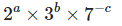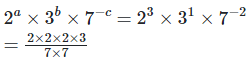= 24/49

21. Simplify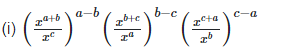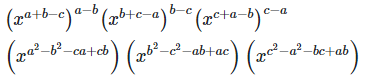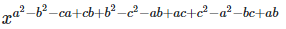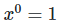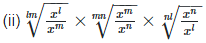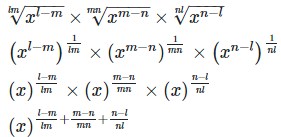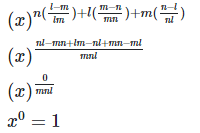22. Show that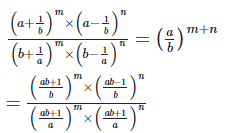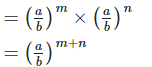Hence LHS = RHS

23.

(i)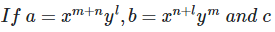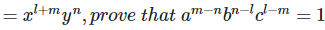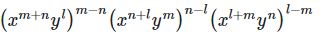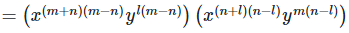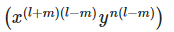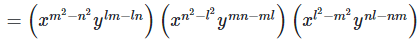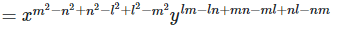= x0 y0

= 1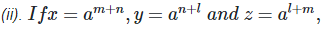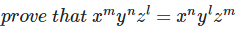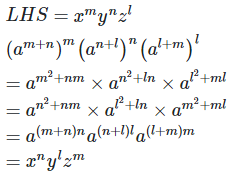Offer running on EduRev: Apply code STAYHOME200 to get INR 200 off on our premium plan EduRev Infinity!

91 docs

,

,

,

,

,

,

,

,

,

,

,

,

,

,

,

,

,

,

,

,

,

,

,

,

,

,

,

,

,

,

,

,

,

;